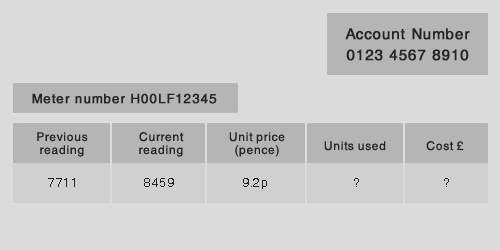## The Electricity Bill

Kilowatt-hours (kWh) is the unit of energy used when calculating the cost of electricity. It is calculated using the equation;

Energy transferred (kilowatt hour, kWh)
=
Power (kilowatts, kW) x Time (hours, h)

Remember a kilowatt is 1000 watts (kilo = 1000)

### Example – Cost of using an electric iron

A 1500W electric iron is used for 30 minutes. What is the cost of electricity if the unit price is 9.5p per kW h?

Power of electric iron = P = 1500W = 1500/1000 = 1.5kW
Time = t = 30min = 30/60 = 0.5 h

Energy transferred = power x time = 1.5 x 0.5 = 0.75 kW h

Cost = 0.75 x 9.5 = 7.125p

### Example – Cost from electricity bill

Calculate the units used and the cost from the electricity bill below?Units used = current reading – previous reading = 8459 – 7711 = 748
Cost = units used x unit price = 748 x 9.2 = 6881.6p

Divide the cost by 100 to convert to pounds = 6881.6/100 = £68.816 = £68.82